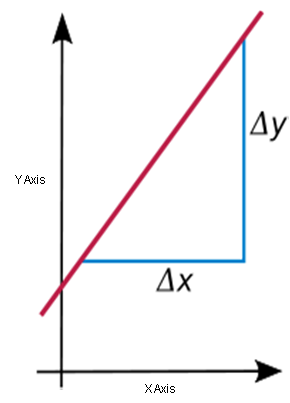A# slopeSlope is sometimes referred to as 'rise over run.' For a straight line in the plane, the slope is the tangent of the angle it forms with the positive x-axis. For a curve, the slope is, by definition, the slope of the tangent line. Therefore, if the slope is constant a line is straight.